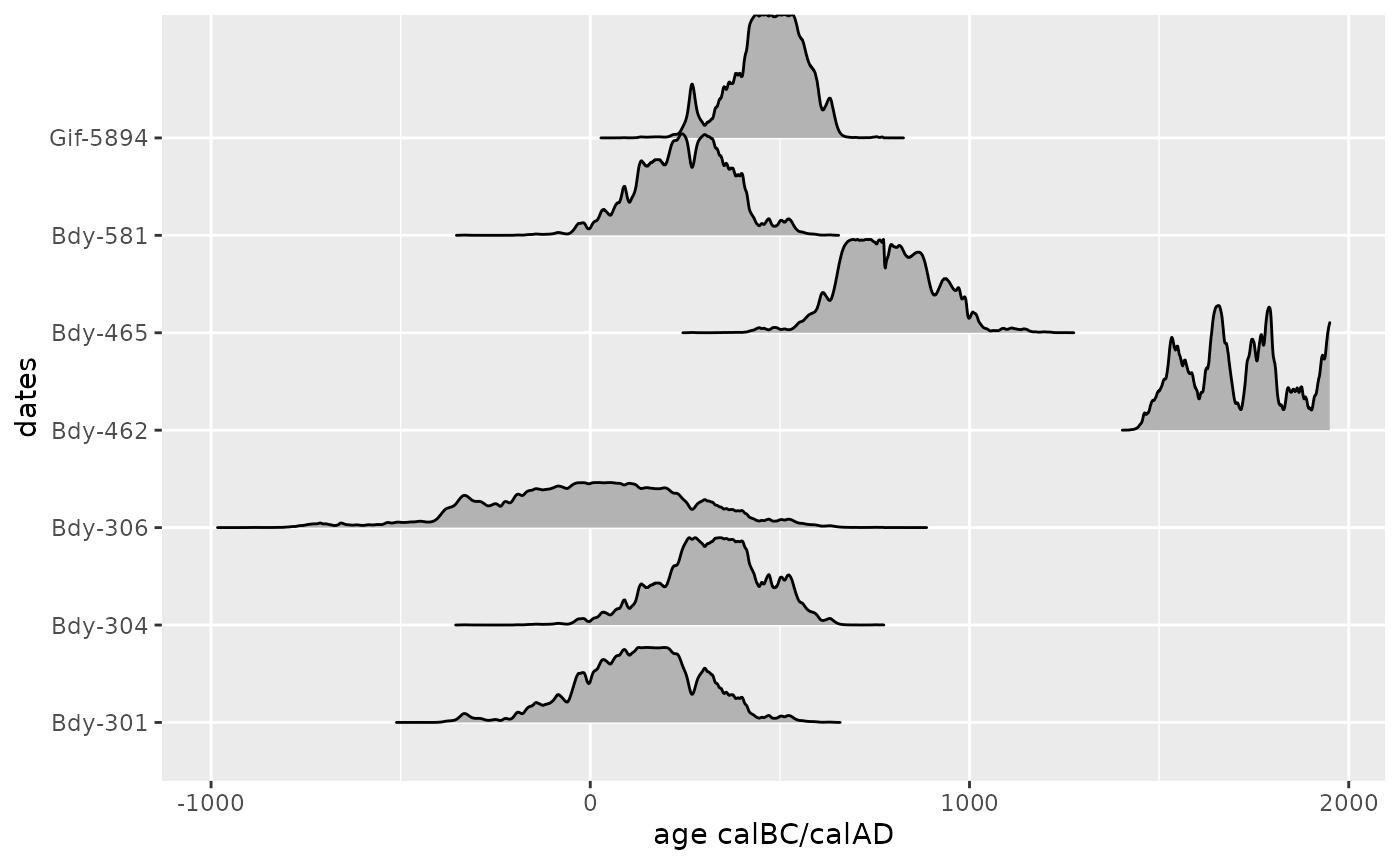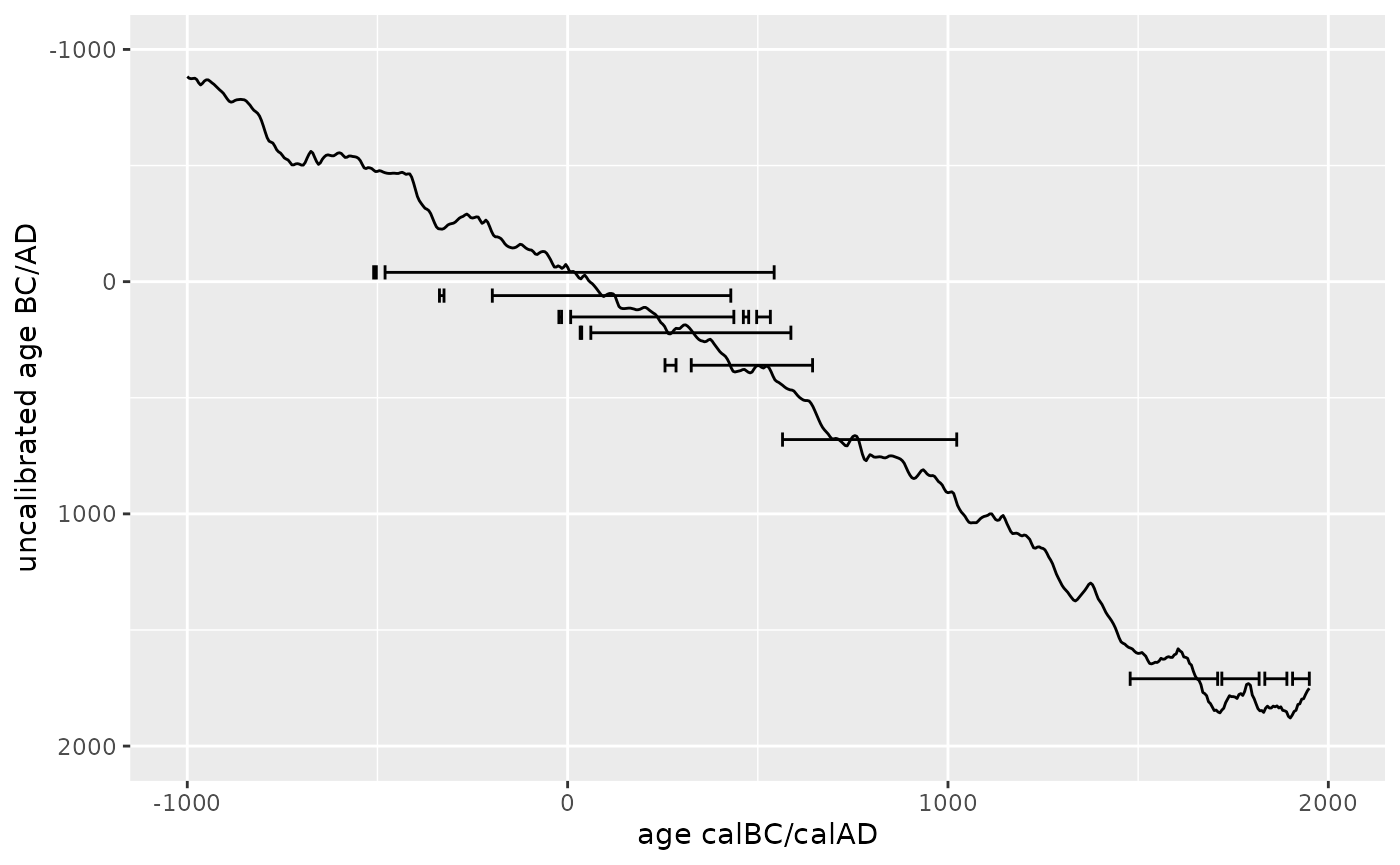This vignette shows some basic workflows to plot radiocarbon dates in `c14_date_list`s. This is only a short compilation to get you started.

So let’s begin by loading the main packages for this endeavour: c14bazAAR and ggplot2. And of course magrittr to enable the pipe (`%>%`) operator. We will use functions from other packages as well, but address them individually with the `::` operator.

``````library(c14bazAAR)
library(ggplot2)
library(magrittr)``````

The basis for this example code is the adrac data collection by Dirk Seidensticker. So let’s download the data with the relevant c14bazAAR getter function:

``adrac <- get_c14data("adrac")``

## Temporal plotting of radiocarbon ages

Radiocarbon dating is a method to determine the absolute age of samples. Therefore one of the main aims for plotting naturally is to display temporal information. Let’s select the dates of one individual site – Batalimo in Central Africa – as a subset to reproduce two of the most common types of radiocarbon date plots.

``````Batalimo <- adrac %>%
dplyr::filter(site == "Batalimo")``````

If age modelling and date plotting on a local or regional scale is the major aim of your analysis, you might want to take a look at the oxcAAR package. It serves as an R interface to OxCal and provides powerful default plotting methods

### Ridgeplots of density distributions

One way to plot radiocarbon ages is to show the probability density distribution of individual calibrated dates as ridgeplots. To produce a plot like that, we first of all need the age-probability information for each date. We can calculate that with the function `c14bazAAR::calibrate()`.

``````Batalimo_calibrated <- Batalimo %>%
calibrate(choices = "calprobdistr")``````

This adds a list column `calprobdistr` to the input `c14_date_list`. The list column contains a nested data.frame for each date with its probability distribution.

``````##  Radiocarbon date list
##  dates: 7
##  sites: 1
##  countries: 1
##  uncalBP: 2000 ― 200
##
## # A data frame: 7 × 17
##   sourcedb sourcedb_version method labnr c14age c14std calprobdistr c13val site
##   <chr>    <date>           <chr>  <chr>  <int>  <int> <list>        <dbl> <chr>
## 1 adrac    2023-09-05       NA     Bdy-…   1890    130 <df>             NA Bata…
## 2 adrac    2023-09-05       NA     Bdy-…   1730    120 <df>             NA Bata…
## 3 adrac    2023-09-05       NA     Bdy-…   1990    210 <df>             NA Bata…
## 4 adrac    2023-09-05       NA     Bdy-…    240     75 <df>             NA Bata…
## 5 adrac    2023-09-05       NA     Bdy-…   1270    125 <df>             NA Bata…
## 6 adrac    2023-09-05       NA     Bdy-…   1798    101 <df>             NA Bata…
## 7 adrac    2023-09-05       NA     Gif-…   1590     90 <df>             NA Bata…
## # ℹ 8 more variables: feature <chr>, period <chr>, material <chr>,
## #   country <chr>, lat <dbl>, lon <dbl>, shortref <chr>, comment <chr>``````

With `tidyr::unnest()` the list column can be dissolved (“unnested”) and integrated into the initial `c14_date_list`. Of course the latter looses its original structure and meaning with this step. Each row now represents the probability for one date and year.

``Batalimo_cal_dens <- Batalimo_calibrated %>% tidyr::unnest(cols = c("calprobdistr"))``

A table like that can be used for plotting a ridgeplot.

``````Batalimo_cal_dens %>%
ggplot() +
# a special geom for ridgeplots is provided by the ggridges package
ggridges::geom_ridgeline(
# the relevant variables that have to be mapped for this geom are
# x (the time -- here the calibrated age transformed to calBC),
# y (the individual lab number of the dates) and
# height (the probability for each year and date)
aes(x = -calage + 1950, y = labnr, height = density),
# ridgeplots lack a scientifically clear y axis for each
# distribution plot and we can adjust the scaling to our needs
scale = 300
) +
ylab("dates")``````### Calcurve plot

Another way to plot radiocarbon dates is to project them onto a calibration curve. The Bchron R package contains a data.frame with the intcal13 calibration curve data. We can load the `intcal13` table directly from Bchron.

``load(system.file('data/intcal13.rda', package = 'Bchron'))``

For this kind of plot it is more convenient to work with the simplified `calrange` output of `c14bazAAR::calibrate()`.

``````Batalimo_calibrated <- Batalimo %>%
calibrate(choices = "calrange")``````

Like the `calprobdistr` option this also adds a list column to the input `c14_date_list`, but a much smaller one. For each date only the age ranges that make up the 2-sigma significance interval of the probability distribution are stored.

``Batalimo_calibrated\$calrange[1:3]``
``````## []
##   dens from   to
## 1 91.9 1521 2148
## 2  3.3 2275 2287
##
## []
##   dens from   to
## 1 93.7 1363 1889
## 2  1.5 1913 1917
##
## []
##   dens from   to
## 1 94.1 1407 2430
## 2  1.2 2453 2460``````

The resulting table can also be unnested to make the list column content available in the main table.

``Batalimo_cal_range <- Batalimo_calibrated %>% tidyr::unnest(cols = c("calrange"))``

Now we can plot the calibration curve and – on top – error bars with the `calrange` sequences.

``````ggplot() +
# line plot of the intcal curve
geom_line(
data = intcal13,
# again we transform the age information from BP to BC
mapping = aes(x = -V1 + 1950, y = -V2 + 1950)
) +
# the errorbars are plotted on top of the curve
geom_errorbarh(
data = Batalimo_cal_range,
mapping = aes(y = -c14age + 1950, xmin = -to + 1950, xmax = -from + 1950)
) +
# we define the age range manually -- typically the calcurve
# is arranged to go from the top left to the bottom right corner
xlim(-1000, 2000) +
ylim(2000, -1000) +## Spatial mapping of radiocarbon dates

Most radiocarbon dates that can be accessed with c14bazAAR have coordinate information for the respective sites where the samples were taken. Spatial maps therefore are an important form of data visualization as well.

`c14_date_list`s can directly be transformed to objects of class `sf`. `sf` objects were introduced by the R package sf which provides a tidy interface to work with spatial data in R.

``adrac_sf <- adrac %>% as.sf()``
``````## Warning in remove_dates_without_coordinates(., quiet): Dates without
## coordinates were removed.``````

This tabular data structure contains the spatial point information for each date in a column geom, but also the initial columns of the input dataset: data.*

``````## Simple feature collection with 2106 features and 3 fields
## Geometry type: POINT
## Dimension:     XY
## Bounding box:  xmin: 8.717 ymin: -16.75 xmax: 30.683 ymax: 12.2
## Geodetic CRS:  WGS 84
## First 10 features:
##    data.labnr data.c14age data.c14std                 geom
## 1    AA-33171       13800         100 POINT (10.078 1.727)
## 2    AA-33172        6665          90 POINT (10.078 1.727)
## 3    AA-33225       10015          55    POINT (28.6 2.17)
## 4    AA-33226        6025          70    POINT (28.6 2.17)
## 5    AA-36757        4254          49 POINT (10.078 1.727)
## 6    AA-36758       10450          93 POINT (10.078 1.727)
## 7    AA-36792        5810         290 POINT (10.078 1.727)
## 8    AA-36793       30300        1300 POINT (10.078 1.727)
## 9    AA-78447        2362          39 POINT (16.924 3.989)
## 10   AA-78448        2171          37 POINT (16.924 3.989)``````

It can be manipulated with the powerful dplyr functions. We `filter` out all dates from one particular publication (Moga 2008), `group` the dates `by` site and apply the `summarise` command to keep only one value per group. As we do not define an operation to fold the other variables in the input table, they are removed. Only the geometry column remains.

``````Moga_spatial <- adrac_sf %>%
dplyr::filter(grepl("Moga 2008", data.shortref)) %>%
dplyr::group_by(data.site) %>%
dplyr::summarise(.groups = "drop")``````

### Interactive map view

The resulting `sf` object can be plotted interactively with the mapview package.

``# Moga_spatial %>% mapview::mapview()``

### Static map plot

The `sf` object can also be used for a static plot – which is useful for publications. We download some simple country border base map vector data with the rnaturalearth R package and transform it to `sf` as well.

``countries <- rnaturalearth::ne_countries() %>% sf::st_as_sf()``
``````## The legacy packages maptools, rgdal, and rgeos, underpinning the sp package,
## which was just loaded, will retire in October 2023.
## Please refer to R-spatial evolution reports for details, especially
## https://r-spatial.org/r/2023/05/15/evolution4.html.
## It may be desirable to make the sf package available;
## package maintainers should consider adding sf to Suggests:.
## The sp package is now running under evolution status 2
##      (status 2 uses the sf package in place of rgdal)``````
``````## Warning: The `returnclass` argument of `ne_download()` sp as of rnaturalearth 1.0.0.
## ℹ Please use `sf` objects with {rnaturalearth}, support for Spatial objects
##   (sp) will be removed in a future release of the package.
## This warning is displayed once every 8 hours.
## Call `lifecycle::last_lifecycle_warnings()` to see where this warning was
## generated.``````

Now we can combine the base layer and our point data to create the prototype of a static map plot.

``````ggplot() +
# geom_sf is a special geom to handle spatial data in the sf format
geom_sf(data = countries) +
# the explicit mapping of variables is not necessary here, as geom_sf
# automatically finds the *geom* column in the input table
geom_sf_text(data = countries, mapping = aes(label = formal_en), size = 2) +
geom_sf(data = Moga_spatial) +
# with geom_sf comes coord_sf to manage the underlying coordinate grid
coord_sf(xlim = c(10, 30), ylim = c(0, 15))``````